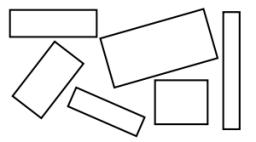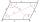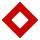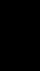# Rectangle 35

Find the area of a rectangle when the diagonal is equal to 30 cms and the width is double the length.

S =  360 cm2

### Step-by-step explanation:Did you find an error or inaccuracy? Feel free to write us. Thank you!Tips to related online calculators
Check out our ratio calculator.
Pythagorean theorem is the base for the right triangle calculator.
Tip: Our volume units converter will help you with the conversion of volume units.

#### You need to know the following knowledge to solve this word math problem:

We encourage you to watch this tutorial video on this math problem:

## Related math problems and questions:

• RectangleThe length of the rectangle are in the ratio 5:12 and the circumference is 238 cm. Calculate the length of the diagonal and area of rectangle.Calculate the volume and surface area of a regular quadrangular prism 35 cm high and the base diagonal of 22 cm.
• Diagonal to areaCalculate the area of a rectangle in which the length of the diagonal is 10 cm.
• Rectangle 3-4-5The sides of the rectangle are in a ratio of 3:4. The length of the rectangle diagonal is 20 cm. Calculate the content of the rectangle.
• RectangleThere is a rectangle with a length of 12 cm and a diagonal 8 cm longer than the width. Calculate the area of a rectangle.
• Uboid volumeCalculate the cuboid volume if the walls are 30cm², 35cm², 42cm²
• Diagonal of the rectangleCalculate the rectangle's diagonal, which area is 54 centimeters square, and the circuit is equal to 30 cm.
• The right triangleThe right triangle ABC has a leg a = 36 cm and an area S = 540 cm2. Calculate the length of the leg b and the median t2 to side b.
• Side and diagonalFind the circumference and the area of the rectangle if given: side a = 8 cm diagonal u = 10 cm.
• Area of iso-trapFind the area of an isosceles trapezoid if the lengths of its bases are 16 cm and 30 cm, and the diagonals are perpendicular to each other.
• Rectangle diagonalThe rectangle, one side of which is 5 cm long, is divided by a 13 cm diagonal into two triangles. Calculate the area of one of these triangles in cm2.
• The diamondThe diamond has sides of 35 cm and the diagonals are in a ratio of 1: 2. Calculate the lengths of the diagonals.
• The sidesThe sides of the rectangle are in a ratio of 3: 5 and its circumference measures 72 cm. Calculate: a) the size of both sides of the rectangle b) the area of the rectangle c) the length of the diagonals
• DiamondSide length of diamond is 35 cm and the length of the diagonal is 56 cm. Calculate the height and length of the second diagonal.
• TrapeziumThe area of trapezium is 35 cm2. Find its altitude if the bases are 6cm and 8 cm.The cone-shaped lampshade has a diameter of 30 cm and a height of 10 cm. How many cm2 of material will we need when we 10% is waste?Find the surface and volume of a cube whose wall diagonal is 5 cm long.